Finance Processes

Stefan is an M&A banker cum startup CFO with deep finance experience accross projects ranging from \$6M series-A raises to \$7Bn LBOs.

## Executive Summary

Why is Python a great programming language for finance professionals to learn?
• Python is a high-level programming language, meaning that it abstracts away and handles many of the technical aspects of programming, such as memory management, that must be explicitly handled in other languages. This makes Python easy to use for those without a technical background.
• Because the language was designed with readability and ease-of-use in mind, it is one of the easiest languages to learn. Python code is concise and close to plain English.
• Python is ideal for prototyping and rapid, iterative development. Its interactive interpreter tools provide environments where you can write and execute each line of code in isolation and see the results immediately.
• At the same time, Python is robust and performant, making it a viable choice also for core systems and larger applications.
• In addition to its large standard library of useful tools, Python has great third-party libraries for financial analysis and computing, such as the Pandas and NumPy libraries used in this tutorial.
What are some use-cases for implementing Python and finance together?
• Python scripts can be used to automate repetitive tasks and workflows, saving time and reducing the risk of manual errors.
• Scripts allow users to easily pull data from spreadsheets, databases, and APIs, or even scrape web data, which then can be processed and analyzed using powerful statistical and analytical tools.
• Various plugins for Excel allow users to create real-time two-way links between your spreadsheets and Python code.
• Python enables new types of analysis, such as Monte Carlo simulations, that are not readily available in standard spreadsheets.
• Algorithmic trading is no longer the exclusive domain of hedge funds and large investment banks. With Python, you can develop, backtest and deploy your own trading strategies in a short time and at a low cost.

For professions that have long relied on trawling through spreadsheets, Python is especially valuable. Citigroup, an American bank, has introduced a crash course in Python for its trainee analysts. - The Economist

Finance professionals have long had access to VBA (Visual Basic for Applications) in Excel to build custom functionality and automate workflows. With the emergence in recent years of Google Sheets as a serious contender in the spreadsheet space, Google Apps Script now offers an additional choice.

However, I would like to draw attention to a third option, the Python programming language, which has become tremendously popular in a number of fields.

In this article, I will provide some examples of what you can accomplish with Python, starting with an overview of the language itself and why it has become so popular in such a wide variety of fields, ranging across web development, machine learning, finance, science, and education, just to name a few. The second half will then consist of a step-by-step tutorial.

The aim of me writing this is to help you decide whether Python looks intriguing enough for you to consider adding it to your financial toolbox. If you take the leap, there are many apps, courses, videos, articles, books and blog posts available to learn the language. At the end of the piece, I have listed some resources that have helped me along the way.

## Use Cases: Examples of What I Have Used Python For

My introduction to programming was learning BASIC on an Oric 1 in the mid-1980s. Back then BASIC was the most common beginner’s language. Other languages that I dabbled with in the late 80s until mid-90s were Pascal and C, but I never used them in any professional capacity, and I didn’t expect to need or use programming skills. To my knowledge at the time in the late 90s, finance and programming were very different fields, when I chose to embark on a career path in finance.

Fast forward to 2012, and I was looking to pick programming back up as a hobby, so I started researching the languages available at the time. It turned out quite a bit had happened, and when I came across Python I was hooked, for many of the reasons that I will outline in the next section. Since then I have used Python for a wide range of tasks, from small scripts to larger projects, both personally and professionally. Many, but not all, have involved spreadsheets, the workbench of many a finance professional.

Here are a few examples of how well spreadsheets and Python can go together:

### 1. Tracking Hundreds of Activities Over Time in an M&A Integration PMO Setup

I work with all aspects of M&A transactions, not just the execution, but also integration. In a recent case, the PMO team decided on a hybrid program and project management approach, using waterfall planning and Gantt charts for high-level plans for each of the twelve integration workstreams, in addition to a Kanban board for tracking the hundreds of activities going on at any given time, in the first 100-day plan and beyond. The Kanban tool that was chosen, MeisterTask, has a number of statistical and reporting features, but our needs went beyond that in terms of analysis and presentation, which required a custom solution. This is the workflow that I automated using Python:

1. Save status of the whole board weekly as a CSV file.
2. Read all historical CSV files into a Pandas DataFrame.
3. Sort, filter, group and manipulate the data into agreed formats of how we want to track progress (by the status of activity, workstream, etc.).
4. Write the output to an Excel file with the data from each analysis within its own sheet, formatted in such a way that it can be simply copied and pasted into think-cell charts.
5. Create tables and charts for the reporting package for the monthly steering committee meeting.

Developing the script required an upfront investment of a few hours, but now, updating the reporting pack for steering committee meetings or ad hoc analysis takes a matter of minutes. Literally, about 30 seconds to go to the right folder and run the script with a one-line command, and then a few minutes to copy-paste the output into the slide deck. With about 500 activities (cards) across twelve workstreams already about a month into execution, weekly tracking of how they move, inside a program timeline of two years, you quickly find yourself dealing with thousands, and eventually tens of thousands of data points across dozens of files. Without automation, we are talking about some very tedious tasks here.

The “time value of money” trade-off between just getting on with things, or adding more initial workload by setting up automation is a common theme in finance. I made a similar decision with the first step of this process, by exporting the data as CSV files. MeisterTask, like many modern web applications, has an API, which can be connected to your Python application, but the time spent setting it up would far outweigh the time savings for our use case here.

So, as you see, oftentimes the optimal solution is to automate certain steps of a workflow and keep others manual.

### 2. Analyzing House Price Statistics Using Web Scraping, Google Maps API, and Excel

Another example is something I did out of personal interest but I want to highlight it because it contains some other interesting elements of Python’s utility:

1. Scrape data of real estate listings, including address, size, number of rooms, asking price, and other features, for a given area; a few hundred to perhaps a thousand lines in total.
2. Save into a Python data structure.
3. Connect to the Google Maps API and, for each listing, retrieve the distance between the property and key landmarks such as the sea, the city center, nearest train station, nearest airport, etc.
4. Export the data to an Excel file.
5. Use standard Excel functionality to run regressions, calculate statistics and create charts on standard metrics such as price per square meter and distance to landmarks.

The results here could be combined with your own personal weightings in terms of preferences, and financial limitations when looking for real estate.

These are only two examples, focused on automating spreadsheet-related work and adding features, but the opportunities with Python are almost endless. In the next section, I will outline the reasons why it has become so popular, before moving on to a step-by-step Monte Carlo simulation tutorial in Python.

## Why Python is a Great Choice for Finance Professionals

The programming language Python has been around since 1990, but it is not until recent years that its popularity has exploded.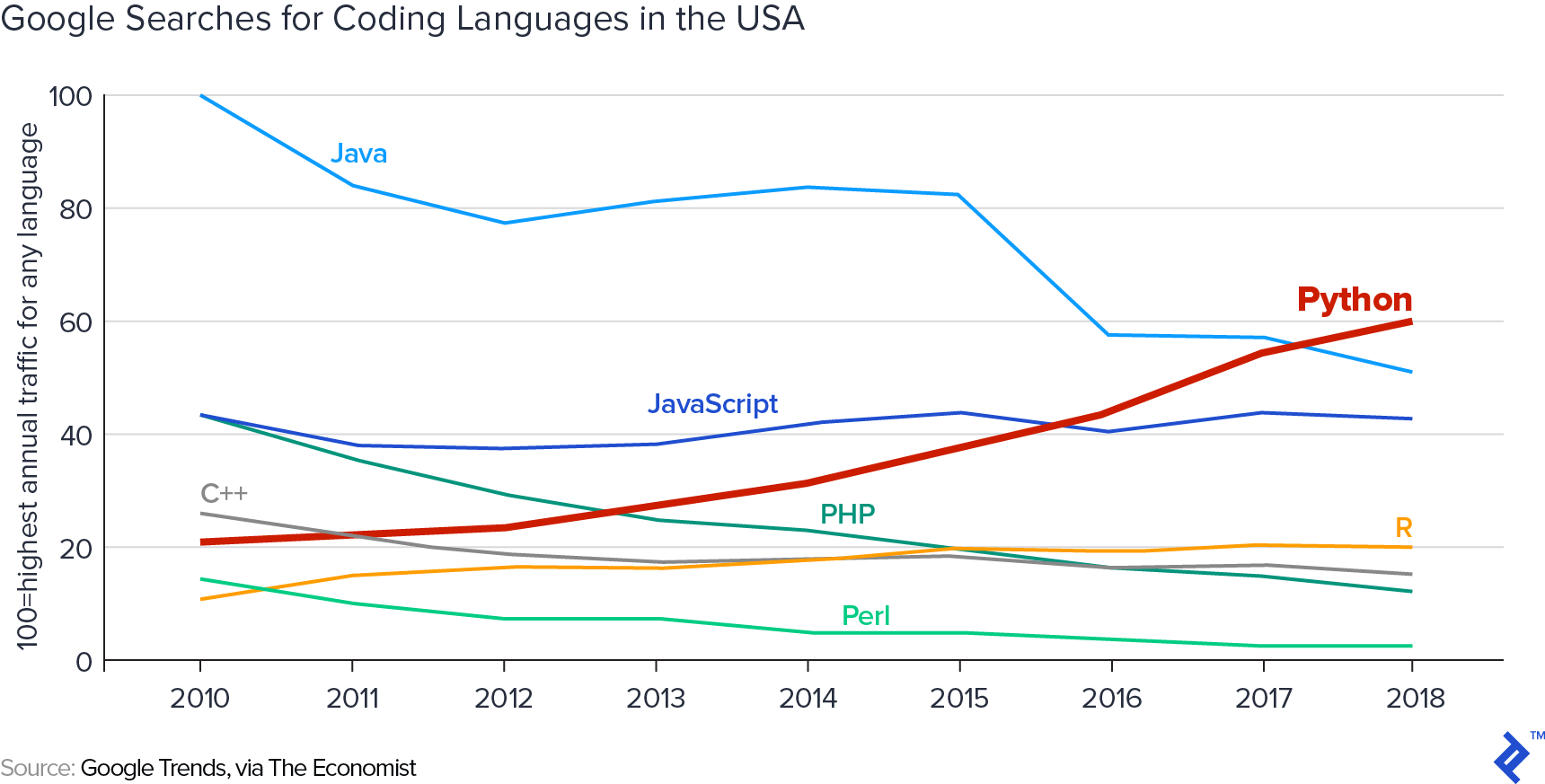There are several reasons for this, let’s look at each in turn.

### 1. Python Is a High-Level Programming Language

A high-level programming language is one that abstracts away many of the details of the inner workings of the computer. A good example is memory management. Lower-level programming languages require a detailed understanding of the complexities of how the computer’s memory is laid out, allocated and released, in addition to the time spent and lines of code required to handle tasks. Python abstracts away and handles many of these details automatically, leaving you to focus on what you want to accomplish.

### 2. It Is Concise

Because Python is a high-level programming language, the code is more concise and almost entirely focused on the business logic of what you want to achieve, rather than technical implementation details. Language design choices contribute to this: as an example, Python doesn’t require the use of curly braces or semicolons to delineate functions, loops, and lines the way many other languages do, which makes it more concise and, as some argue, improves readability.

### 3. Easy to Learn and Understand

One observation that has influenced language design choices in Python is that programs are read more often than they are written. Python excels here as its code looks very close to plain English, especially if you name the different components of your script or program in a sensible manner.

### 4. Suitable for Rapid, Iterative Development

Enlightened trial and error outperforms the planning of flawless intellects. - David Kelley

Python is ideal for prototyping and rapid, iterative development (and, yes, trial-and-error) because interactive interpreter tools such as the Python shell, IPython, and Jupyter notebooks are front and center in the Python toolchain. In these interactive environments, you can write and execute each line of code in isolation and see the results (or a helpful error message) immediately. Other languages have this too, but in most cases not to the same degree as Python.

### 5. Can Be Used Both for Prototyping and Production Code

In addition to being great for prototyping, Python is also an excellent and powerful language for large production applications. Some of the largest software companies in the world make heavy use of Python in a variety of applications and use cases.

### 6. Comes with “Batteries Included:” The Python Standard Library

Everything needed for basic operations is built right into the language, but in addition to that, the Python standard library has tools for working with files, media, networking, date and time information, and much more. This allows you to accomplish a wide variety of tasks without having to look for third-party packages.

### 7. Great Third-party Libraries for Financial Analysis

For finance professionals, Pandas with its DataFrame and Series objects, and Numpy with its ndarray are the workhorses of financial analysis with Python. Combined with matplotlib and other visualization libraries, you have great tools at your disposal to assist productivity.

### 8. Python Is Free!

Python is developed under an open source license making it free also for commercial use.

## Step-by-step Tutorial of Using Python and Finance Together

What follows is a step-by-step tutorial showing how to create a simplified version of the Monte Carlo simulation described in my previous blog post, but using Python instead of the @RISK plugin for Excel.

Monte Carlo methods rely on random sampling to obtain numerical results. One such application is to draw random samples from a probability distribution representing uncertain potential future states of the world where variables or assumptions can take on a range of values.

It is helpful to do the Monte Carlo simulation on a simplified DCF valuation model instead of the more common examples you see showing valuation of options or other derivatives, since for this we don’t need any math beyond the basics of calculating the financial statements and discounting cash flows, allowing us to focus on the Python concepts and tools. Please note though that this basic tutorial model is meant to illustrate the key concepts, and is not useful as-is for any practical purposes. I also won’t touch on any of the more academic aspects of Monte Carlo simulations.

The tutorial assumes that you are familiar with the basic building blocks of programming, such as variables and functions. If not, it might be helpful to take 10 minutes to check the key concepts in for example this introduction.

### The Starting Point and Desired Outcome

I start with the same very simplified DCF valuation model used in the Monte Carlo simulation tutorial. It has some key line items from the three financial statements, and three highlighted input cells, which in the Excel version have point estimates that we now want to replace with probability distributions to start exploring potential ranges of outcomes.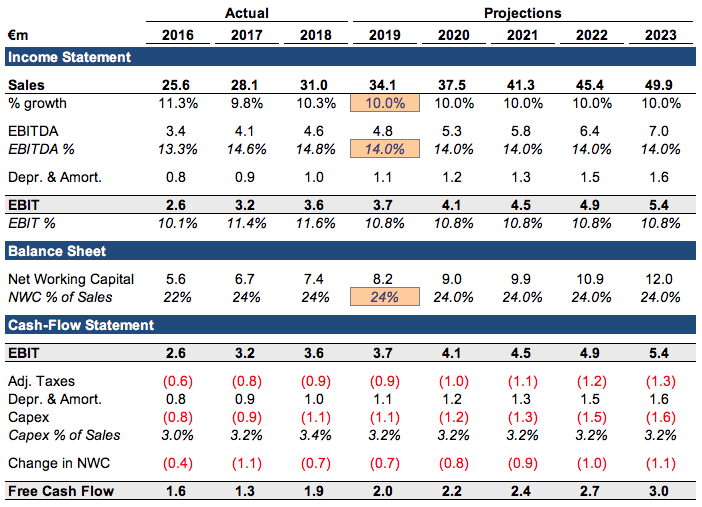### A Two-Step Approach to Developing a Small Script

Make it work, make it right, make it fast - Kent Beck

The intention of this tutorial is to give finance professionals new to Python an introduction not only to what a useful program might look like, but an introduction also to the iterative process you can use to develop it. It, therefore, has two parts:

1. First, I develop a working prototype using a straightforward approach which I think is easy to follow and not completely unlike the process one could use to start this project if you were to start from scratch.
2. Then, after having developed the working prototype, I walk through the process of refactoring - changing the structure of code without changing its functionality. You may want to stick around for that part - it is a more elegant solution than the first one, and, as a bonus, it is about 75x faster in terms of execution time.

## 1. Developing a Working Prototype

### Setting up the Jupyter Notebook

The Jupyter notebook is a great tool for working with Python interactively. It is an interactive Python interpreter with cells that can contain code, Markdown text, images, or other data. For this tutorial I used the Python Quant Platform, but I can also recommend Colaboratory by Google, which is free and runs in the cloud. Once there, simply select “New Python 3 Notebook” in the “File” menu, and you are ready to go.

Having done that, the next step is to import the third-party packages we need for data manipulation and visualizations, and tell the program that we want to see charts inline in our notebook, instead of in separate windows:

``````import numpy as np
import pandas as pd
import matplotlib.pyplot as plt
%matplotlib inline
``````

A note before we start naming our first variables. As I already highlighted, readability is one of Python’s strengths. Language design goes a long way to support that, but everyone writing code is responsible for making it readable and understandable, not only for others but also for themselves. As Eagleson’s Law states, “Any code of your own that you haven’t looked at for six or more months might as well have been written by someone else.”

A good rule of thumb is to name the components of your program in such a way that you minimize the need for separate comments that explain what your program does.

With that in mind, let’s move on.

### Creating the Financial Statements

There are many ways that we can work with existing spreadsheet data in Python. We could, for example, read a sheet into a Pandas DataFrame with one line of code using the `read_excel` command. If you want a tighter integration and real-time link between your spreadsheet and Python code, there are both free and commercial options available to provide that functionality.

Since the model here is very simple, and to focus us on the Python concepts, we will be recreating it from scratch in our script. At the end of the first part, I will show how you can export what we have created to a spreadsheet.

As a first step towards creating a Python representation of the financial statements, we will need a suitable data structure. There are many to choose from, some built into Python, others from various libraries, or we can create our own. For now, let’s use a Series from the Pandas library to have a look at its functionality:

``````years = ['2018A', '2019B', '2020P', '2021P', '2022P', '2023P']
sales = pd.Series(index=years)
sales['2018A'] = 31.0
sales
``````

This input and its corresponding output is shown below: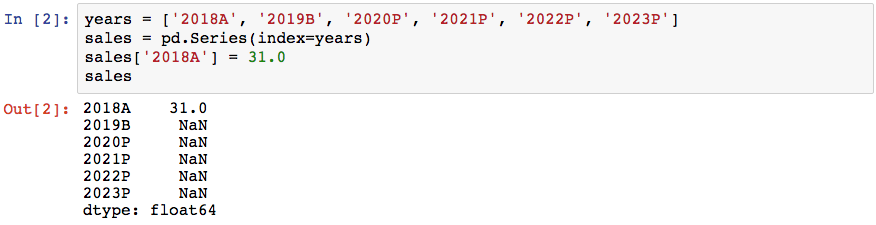With the first three lines we have created a data structure with an index consisting of years (each marked to show if it is Actual, Budget or Projected), a starting value (in millions of euros, as in the original DCF model), and empty (NaN, “Not a Number”) cells for the projections. The fourth line prints a representation of the data - in general, typing the name of a variable or other objects in the interactive interpreter will usually give you a sensible representation of it.

Next, we declare a variable to represent the projected annual sales growth. At this stage, it is a point estimate, the same figure as in our original DCF model. We want to first use those same inputs and confirm that our Python version performs the same and gives the same result as the Excel version, before looking at replacing point estimates with probability distributions. Using this variable, we create a loop that calculates the sales in each year of the projections based on the previous year and the growth rate:

``````growth_rate = 0.1
for year in range(1, 6):
sales[year] = sales[year - 1] * (1 + growth_rate)

sales
``````

We now have projected sales, instead of NaN: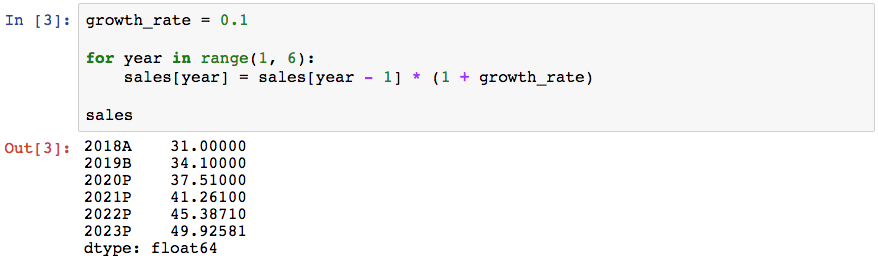Using the same approach, we continue through the financial statements, declaring variables as we need them and performing the necessary calculations to eventually arrive at free cash flow. Once we get there we can check that what we have corresponds to what the Excel version of the DCF model says.

``````ebitda_margin = 0.14
depr_percent = 0.032
ebitda = sales * ebitda_margin
depreciation = sales * depr_percent
ebit = ebitda - depreciation
nwc_percent = 0.24
nwc = sales * nwc_percent
change_in_nwc = nwc.shift(1) - nwc
capex_percent = depr_percent
capex = -(sales * capex_percent)
tax_rate = 0.25
tax_payment = -ebit * tax_rate
tax_payment = tax_payment.apply(lambda x: min(x, 0))
free_cash_flow = ebit + depreciation + tax_payment + capex + change_in_nwc
free_cash_flow
``````

This gives us the free cash flows: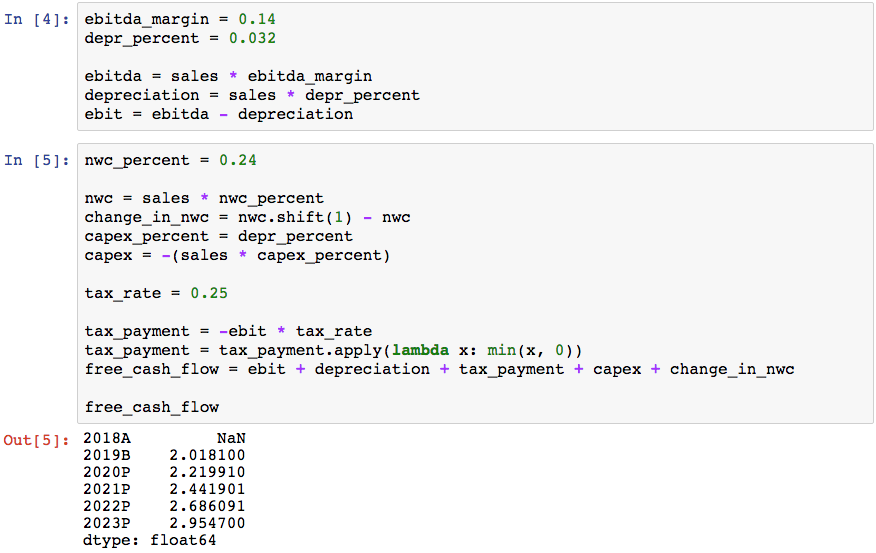The one line above that perhaps needs a comment at this stage is the second `tax_payment` reference. Here, we apply a small function to ensure that in scenarios where profit before tax becomes negative, we won’t then have a positive tax payment. This shows how effectively you can apply custom functions to all cells in a Pandas Series or DataFrame. The actual function applied is, of course, a simplification. A more realistic model for a larger valuation exercise would have a separate tax model that calculates actual cash taxes paid based on a number of company-specific factors.

### Performing the DCF Valuation

Having arrived at projected cash flows, we can now calculate a simple terminal value and discount all cash flows back to the present to get the DCF result. The following code introduces indexing and slicing, which allows us to access one or more elements in a data structure, such as the Pandas Series object.

We access elements by writing square brackets directly after the name of the structure. Simple indexing accesses elements by their position, starting with zero, meaning that `free_cash_flow` would give us the second element. `[-1]` is shorthand for accessing the last element (the last year’s cash flow is used to calculate the terminal value), and using a colon gives us a slice, meaning that `[1:]` gives us all elements except the first one, since we don’t want to include the historical year `2018A` in our DCF valuation.

``````cost_of_capital = 0.12
terminal_growth = 0.02
terminal_value = ((free_cash_flow[-1] * (1 + terminal_growth)) /
(cost_of_capital - terminal_growth))
discount_factors = [(1 / (1 + cost_of_capital)) ** i for i in range (1,6)]
dcf_value = (sum(free_cash_flow[1:] * discount_factors) +
terminal_value * discount_factors[-1])
dcf_value
``````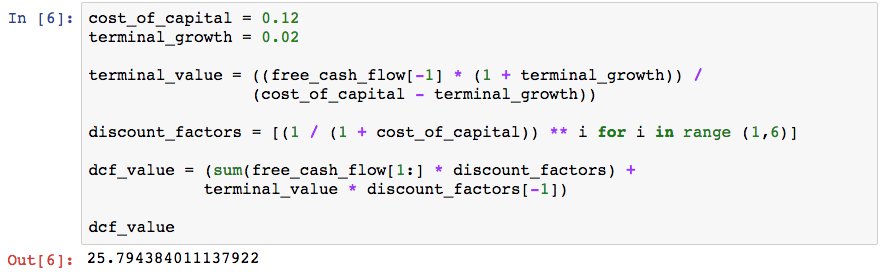That concludes the first part of our prototype - we now have a working DCF model, albeit a very rudimentary one, in Python.

### Exporting the Data

Before moving on to the actual Monte Carlo simulation, this might be a good time to mention the exporting capabilities available in the Pandas package. If you have a Pandas DataFrame object, you can write that to an Excel file with one line using the `to_excel` method. There is similar functionality to export to more than a dozen other formats and destinations as well.

``````output = pd.DataFrame([sales, ebit, free_cash_flow],
index=['Sales', 'EBIT', 'Free Cash Flow']).round(1)
output.to_excel('Python DCF Model Output.xlsx')
output
``````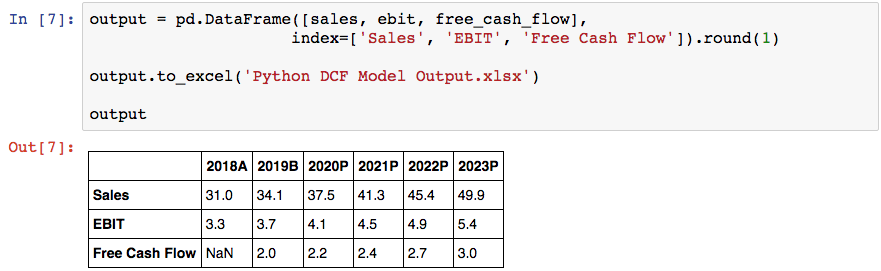### Creating Probability Distributions for Our Monte Carlo Simulation

Now we are ready to tackle the next challenge: to replace some of the point estimate inputs with probability distributions. While the steps up to this point may have seemed somewhat cumbersome compared to building the same model in Excel, these next few lines will give you a glimpse of how powerful Python can be.

Our first step is to decide how many iterations we want to run in the simulation. Using 1,000 as a starting point strikes a balance between getting enough data points to get sensible output plots, versus having the simulation finish within a sensible time frame. Next, we generate the actual distributions. For the sake of simplicity, I generated three normal distributions here, but the NumPy library has a large number of distributions to choose from, and there are other places to look as well, including the Python standard library. After deciding which distribution to use, we need to specify the parameters required to describe their shape, such as mean and standard deviation, and the number of desired outcomes.

``````iterations = 1000
sales_growth_dist = np.random.normal(loc=0.1, scale=0.01, size=iterations)
ebitda_margin_dist = np.random.normal(loc=0.14, scale=0.02, size=iterations)
nwc_percent_dist = np.random.normal(loc=0.24, scale=0.01, size=iterations)
plt.hist(sales_growth_dist, bins=20)
plt.show()
``````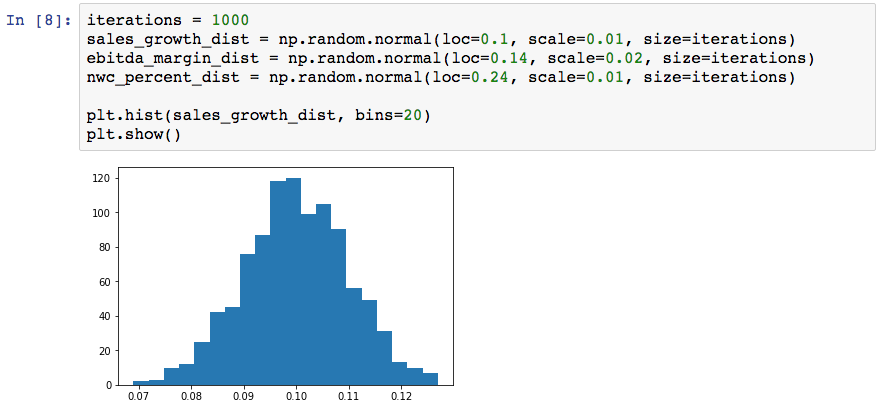Here you could argue that EBITDA should not be a separate random variable independent from sales but instead correlated with sales to some degree. I would agree with this, and add that it should be driven by a solid understanding of the dynamics of the cost structure (variable, semi-variable and fixed costs) and the key cost drivers (some of which may have their own probability distributions, such as for example input commodities prices), but I leave those complexities aside here for the sake of space and clarity.

The less data you have to inform your choice of distribution and parameters, the more you will have to rely on the outcome of your various due diligence workstreams, combined with experience, to form a consensus view on ranges of likely scenarios. In this example, with cash flow projections, there will be a large subjective component, which means that visualizing the probability distributions becomes important. Here, we can get a basic visualization, showing the sales growth distribution, with only two short lines of code. This way we can quickly view any distribution to eyeball one that best reflects the team’s collective view.

Now we have all the building blocks we need to run the simulation, but they are not in a convenient format for running the simulation. Here is the same code we have worked with thus far but all gathered in one cell and rearranged into a function for convenience:

``````def run_mcs():

# Create probability distributions
sales_growth_dist = np.random.normal(loc=0.1, scale=0.01, size=iterations)
ebitda_margin_dist = np.random.normal(loc=0.14, scale=0.02, size=iterations)
nwc_percent_dist = np.random.normal(loc=0.24, scale=0.01, size=iterations)

# Calculate DCF value for each set of random inputs
output_distribution = []
for i in range(iterations):
for year in range(1, 6):
sales[year] = sales[year - 1] * (1 + sales_growth_dist)
ebitda = sales * ebitda_margin_dist[i]
depreciation = (sales * depr_percent)
ebit = ebitda - depreciation
nwc = sales * nwc_percent_dist[i]
change_in_nwc = nwc.shift(1) - nwc
capex = -(sales * capex_percent)
tax_payment = -ebit * tax_rate
tax_payment = tax_payment.apply(lambda x: min(x, 0))
free_cash_flow = ebit + depreciation + tax_payment + capex + change_in_nwc

# DCF valuation
terminal_value = (free_cash_flow[-1] * 1.02) / (cost_of_capital - 0.02)
free_cash_flow[-1] += terminal_value
discount_factors = [(1 / (1 + cost_of_capital)) ** i for i in range (1,6)]
dcf_value = sum(free_cash_flow[1:] * discount_factors )
output_distribution.append(dcf_value)

return output_distribution
``````

We can now run the whole simulation and plot the output distribution, which will be the discounted cash flow value of this company in each of the 1,000 iterations, with the following code. The `%time` command is not Python code but a notebook shorthand that measures the time to run something (you could instead use the Python function from the standard library). It depends on the computer you run it on, but this version needs 1-2 seconds to run the 1,000 iterations and visualize the outcome.

``````%time plt.hist(run_mcs(), bins=20, color='r')
plt.show()
``````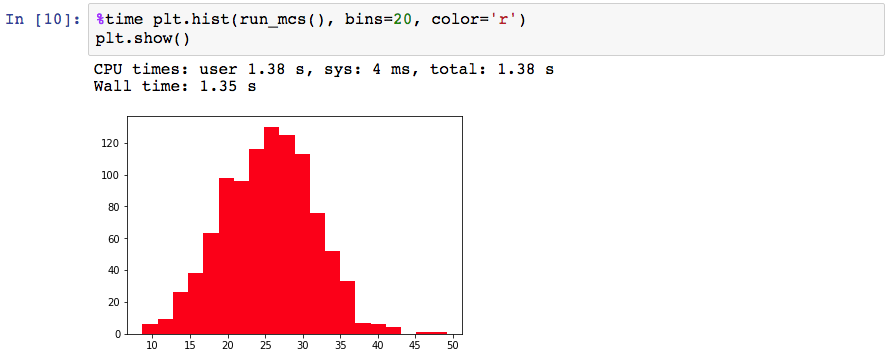## 2. Refining the Prototype

The lurking suspicion that something could be simplified is the world’s richest source of rewarding challenges. - Edsger Dijkstra

Refactoring refers to the process of rewriting existing code to improve its structure without changing its functionality, and it can be one of the most fun and rewarding elements of coding. There can be several reasons to do this. It might be to:

1. Organize the different parts in a more sensible way.
2. Rename variables and functions to make their purpose and workings clearer.
3. Allow and prepare for future features.
4. Improve the execution speed, memory footprint or other resource utilization.

To show what one step in that process might look like, I cleaned up the prototype that we just walked through by collecting all initial variables in one place, rather than scattered throughout as in the prototype script, and optimized its execution speed through a process called vectorization.

Using NumPy arrays enables you to express many kinds of data processing tasks as concise array expressions that might otherwise require writing loops. This practice of replacing explicit loops with array expressions is commonly referred to as vectorization. Wes McKinney

It now looks cleaner and easier to understand:

``````# Key inputs from DCF model
years = 5
starting_sales = 31.0
capex_percent = depr_percent = 0.032
sales_growth = 0.1
ebitda_margin = 0.14
nwc_percent = 0.24
tax_rate = 0.25
# DCF assumptions
r = 0.12
g = 0.02
# For MCS model
iterations = 1000
sales_std_dev = 0.01
ebitda_std_dev = 0.02
nwc_std_dev = 0.01
``````
``````def run_mcs():

# Generate probability distributions
sales_growth_dist = np.random.normal(loc=sales_growth,
scale=sales_std_dev,
size=(years, iterations))
ebitda_margin_dist = np.random.normal(loc=ebitda_margin,
scale=ebitda_std_dev,
size=(years, iterations))
nwc_percent_dist = np.random.normal(loc=nwc_percent,
scale=nwc_std_dev,
size=(years, iterations))

# Calculate free cash flow
sales_growth_dist += 1
for i in range(1, len(sales_growth_dist)):
sales_growth_dist[i] *= sales_growth_dist[i-1]
sales = sales_growth_dist * starting_sales
ebitda = sales * ebitda_margin_dist
ebit = ebitda - (sales * depr_percent)
tax = -(ebit * tax_rate)
np.clip(tax, a_min=None, a_max=0)
nwc = nwc_percent_dist * sales
starting_nwc = starting_sales * nwc_percent
prev_year_nwc = np.roll(nwc, 1, axis=0)
prev_year_nwc = starting_nwc
delta_nwc = prev_year_nwc - nwc
capex = -(sales * capex_percent)
free_cash_flow = ebitda + tax + delta_nwc + capex
# Discount cash flows to get DCF value
terminal_value = free_cash_flow[-1] * (1 + g) / (r - g)
discount_rates = [(1 / (1 + r)) ** i for i in range (1,6)]
dcf_value = sum((free_cash_flow.T * discount_rates).T)
dcf_value += terminal_value * discount_rates[-1]

return dcf_value
``````

The main difference you will notice between this version and the previous one is the absence of the `for i in range(iterations)` loop. Using NumPy’s array operation, this version runs in 18 milliseconds compared to the 1.35 seconds for the prototype version - roughly 75x faster.

``````%time plt.hist(run_mcs(), bins=20, density=True, color="r")
plt.show()
``````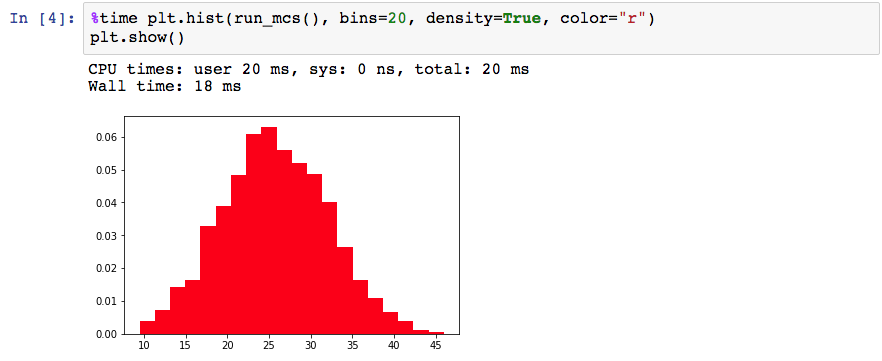I’m sure that further optimization is possible, since I put together both the prototype and refined version in a short time solely for the purpose of this tutorial.

### Taking it Further

This tutorial showed some of the powerful features of Python, and if you were to develop this further the opportunities are almost endless. You could for example:

• Scrape or download relevant company or sector statistics from web pages or other data sources, to help inform your choice of assumptions and probability distributions.
• Use Python in quantitative finance applications, such as in an automated trading algorithm based on fundamental and/or macroeconomic factors.
• Build exporting capabilities that generate output in a spreadsheet and/or presentation format, to be used as part of your internal transaction review and approval process, or for external presentations.

I haven’t even touched upon what you could also do with the various web, data science, and machine learning applications that have contributed to Python’s success.

## In Summary: A Useful Language for Your Financial Toolbox

This article gave an introduction to the Python programming language, listed some of the reasons why it has become so popular in finance and showed how to build a small Python script. In a step-by-step tutorial, I walked through how Python can be used for iterative prototyping, interactive financial analysis, and for application code for valuation models, algorithmic trading programs and more.

For me, at the end of the day, the killer feature of Python technology is that it is simply fun to work with! If you enjoy problem-solving, building things and making workflows more efficient, then I encourage you to try it out. I would love to hear what you have done with it or would like to do with it.

## Understanding the basics

### How is Python used in finance?

Python is mostly used for quantitative and qualitative analysis for asset price trends and predictions. It also lends itself well to automating workflows across different data sources.

### When was Python created?

Python was conceived in the 1980s and first implemented in December 1989.

### What is Python programming used for?

Python is a malleable, generalist programming language with use cases across a range of fields. By taking care of the programming aspect of the application, it allows the programmer to focus on the functionality of their creation.